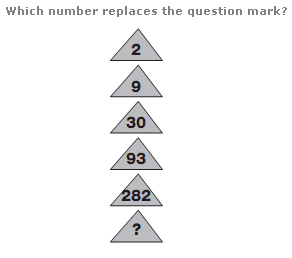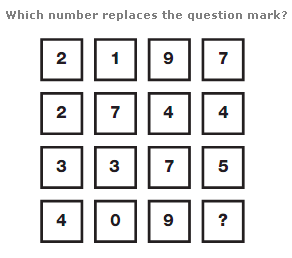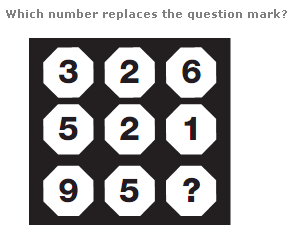# Puzzles - Number puzzles

### Exercise :: Number puzzlesAnswer : 849 Explanation : Working from top to bottom, add 1 to each number and multiply by 3 to give the next value down.Answer : 6 Explanation : Working in rows, from top to bottom, and reading each row as a 4 digit number, rows follow the sequence of Cube Numbers, from 13 to 16.Answer : 8 Explanation : Starting in the top left and working in columns, add the first two numbers together and add 1 to give the number at the bottom of each row.